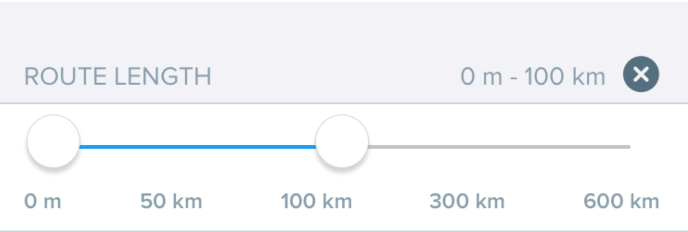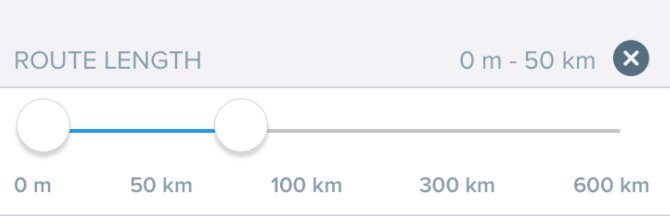# Non linear slider

I have been to implement a part of it (without f(0.25) = 50, f(0.75) = 300) using exp/log ; a bit confused about wanting to implement it with the following constraints; any pointers?

f(0.0) = 0
f(0.25) = 50
f(0.5) = 100
f(0.75) = 300
f(1.0) = 600

looking for a representative function with the constants of it constrained so that I can have five equations with five unknowns or something of that nature - I thinkHi @lganti,
Your image and the question are a bit out of sync. What is it that you want to implement? Do you want to know the distances when you select a segment (using 2 sliders)? If you change your range to a max of 1000 then the formula that you are seeking will not work.

The easiest way to manage what you have in the image is an array of the distances, that will give you a way to display them too and a slider that has values 1-4, so you know which array slot to get the value from and subtract accordingly to get the route length.

If your question is something else, please try to reiterate it better

cheers,

Currently have a linear slider with [0, 150, 300, 450, 600+] but looking to change it to a non-linear one like [0, 50, 100, 300, 600+] to make it more user friendly as most of the users tend to look up objects < 300km or so; I could change the max to 300 and have a linear slider but that is my plan B;

I implemented a non-linear scale with the following constraints:

• f(0) = 0
• f(0.5) = 100
• f(1) = 600

and ended up with an equation equating displayValue and sliderValue but I want the displayValues to be spaced as shown in the image (if you look at the image with 0 m - 50km, the point where the circle is - is indeed 50km as I implemented an exp/log scale)

but I want to, I think, have a linear scale [0, 50, 100] before 100 and after 100 i.e. [100, 300, 600] or something of that nature

hope the above is a better description than the original